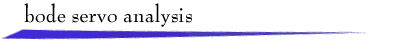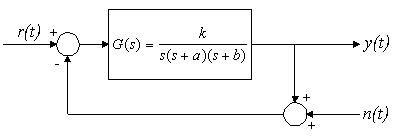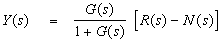A unity-feedback system with third-order plant is driven by a reference signal r(t) and a sensor noise signal n(t). Typical performance objectives involve tracking the reference signal while rejecting the influence of the sensor noise.

The Laplace transform description of the closed-loop system is:Shown below are the open-loop Bode magnitude and phase plots with the corner frequencies a and b marked on the magnitude plot and the gain and phase crossover frequencies labeled. The left mouse button can be used to drag corner frequencies to desired locations, thereby adjusting the values of a, b, and k. The corresponding closed-loop Bode magnitude plot is also shown, with the closed-loop bandwidth labeled.

A selection of reference inputs is available, and high-frequency sensor noise can be selected at two amplitude levels. Plots of r(t) and y(t) are shown, and the Table button provides numerical values of important system parameters and response characteristics. (Rise time is the 10% - 90% rise time. Mean-square steady-state error is computed on the range 15 < t < 25.)

Sample Exercise on Closed-Loop Bandwidth: With no sensor noise, adjust the open-loop gain and corner frequencies so that the step response exhibits overshoot no greater than 20% and a rise time no greater than 0.2 sec. Note the value of mean-square steady-state error in the Table. Then select low-gain sensor noise and adjust the open-loop parameters to achieve mean-square, steady-state error no greater than 0.0020 with 20% overshoot bound and rise time as small as possible.

Sample Exercise on Stability Margins: Fix one of the open-loop corner frequencies at  a = 2 rad/sec.  Adjust the other corner frequency (thereby adjusting b and k ) to obtain a step response with rise time less than 0.6 sec, gain margin at least 6 db, and phase margin at least 35 deg.

Sample Exercise on Closed-Loop Frequency Response:  Adjust the open-loop corner frequencies and gain so that the closed-loop frequency response has the following properties:  the maximum magnitude is 0 db,  the 3 db  bandwidth Wbw = 2 rad/sec, and the closed-loop magnitude at 8 rad/sec is less than  - 20 db.return to demonstrations page

 Applet by Steven Crutchfield.